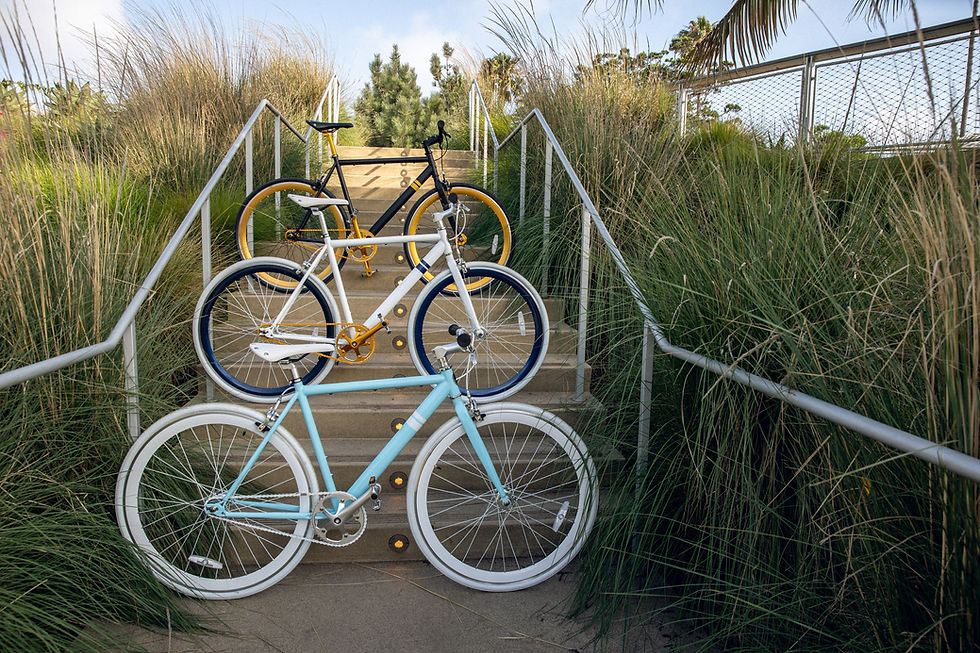top of pageSearch

# What is cross-validation? How can we validate a machine learning model using cross-validation?When we build a machine learning model, it is really important to find a way to validate that the model we built and the hyperparameters used are a good fit for the data. Model validation may sound very simple: after selecting the model and choosing the hyperparameters to build the model, we can estimate how effective the model is by applying it to some test data and comparing the predicted values with the known values. But there are some pitfalls which must be avoided.

Before exploring cross-validation to validate a model, let’s try to use a naive approach to model validation and see how it fails.

### Model validation the wrong way

Let’s use the Iris dataset and k-neighbors classifier to demonstrate this. Let’s begin by importing the required libraries from Scikit-Learn:

```from sklearn.datasets import load_iris
from sklearn.neighbors import KNeighborsClassifier
from sklearn.metrics import accuracy_score```

`iris = load_iris()`
```x = iris.data
y = iris.target```

We’ll use the k-neighbors classifier with n_neighbors=1. This means that the label of an unknown data point is the same as the closest training point label.

`clf_model = KNeighborsClassifier(n_neighbors=1)`

Then we will train the model and predict the labels for data that we already know:

```clf_model.fit(x,y)
y_pred = clf_model.predict(x)```

Finally, we will find out the accuracy of the model prediction:

`accuracy_score(y, y_pred)`
```Output:
1.0```

We can see that the accuracy score is 1.0, which means that 100% of the labels have been correctly predicted for the points. But in realtime, it is impossible to get 100% accuracy from a model.

In fact, there are few fundamental flaws in this approach:

1. The same data is used to train and evaluate the model

2. KNN model is an instance-based model that stores the training data and compares the stored labels to predict the labels for new data. So, this model will always give 100% accuracy every time, except few cases.

So, what is the right way? Model validation can be done using holdout sets and cross-validation.

### Model validation using holdout sets

Holdout sets are nothing but, we hold back a subset of the dataset and use the remaining data for training the model and then use the subset that was held back to evaluate the model. Basically, the whole dataset is split into training and testing sets. We can split the dataset using the train_test_split function in Scikit-Learn:

`from sklearn.model_selection import train_test_split`
```# Splitting the dataset into train and test with 50% in each set
x_train, x_test, y_train, y_test = train_test_split(x,y,
test_size = 0.5, random_state = 0)```

Fit the model using the training set:

```#fit the model on training set
clf_model.fit(x_train,y_train)```

Finally, evaluate the model and find out the accuracy score for this approach.

```# Evaluate the model using the test set and find the accuracy
y_pred = clf_model.predict(x_test)
accuracy_score(y_test, y_pred)```
```Output:
0.9066666666666666```

We can see that with this approach, the KNN classifier model is 90% accurate which is reasonable. In this approach, the model has not seen the holdout set which is like totally unknown data for the model.

The disadvantage with holdout sets: half the dataset did not contribute to the training of the model. This can cause problems when the training data is small.

One way to address this is to use cross-validation: which means we do a sequence of fits on the same model where each subset is used both as a training set and as a validation set.Image by Author: Visualization of two-fold cross-validation

As shown in the above figure, we will do two validation trials, alternately using each subset of the data as the holdout set.

```pred1 = clf_model.fit(x_train,y_train).predict(x_test)
pred2 = clf_model.fit(x_test,y_test).predict(x_train)```
`accuracy_score(y_test, pred1), accuracy_score(y_train, pred2)`
```Output:
(0.9066666666666666, 0.96)```

We can then take a mean of these 2 accuracy scores to measure the model performance. This type of cross-validation is called two-fold cross-validation.

We can also expand on the number of trials with more fold in the data, as shown in the below figure.Image by Author: Visualization of five-fold cross-validation

Here we have split the data into five sets and use each one of them, in turn, to evaluate the model and fit on the other 4/5 of the data. Scikit-Learn provides a function called cross_val_score to perform such cross-validations:

```from sklearn.model_selection import cross_val_score
scores = cross_val_score(clf_model,x,y,cv=5)
#cv =5 denotes no.of trials
print(scores)```
```Output:
array([0.96666667, 0.96666667, 0.93333333, 0.93333333, 1.        ])```

We get an array of accuracy scores for the five trials as shown above. we can then calculate the mean of these 5 values to get the final accuracy score:

```scores.mean()
Output:
0.96```

Scikit-Learn provides various cross-validation schemes that can be useful in different situations. For example, we can have the folds equal to the number of data points; that is, we train the model with all points but one in each trial. This type of cross-validation is called leave-one-out cross-validation and can be used as shown below:

```from sklearn.model_selection import LeaveOneOut
scores = cross_val_score(clf_model,x,y,cv=LeaveOneOut())
scores```Image by Author: Output of leave-one-out cross-validation code

Since the iris dataset has 150 samples, the leave-one-out cross-validation yields the accuracy score for 150 trials, and the score indicates either successful (1.) or unsuccessful (0.) prediction. The mean of these scores will give us the estimate to measure the model performance as shown below:

```scores.mean()

Output:
0.96```

Similarly, other cross-validation schemes can be used to evaluate any model performance.

To explore more on Scikit-Learn‘s cross-validation iterators, please review Scikit-Learn’s online documentation. (https://scikit-learn.org/stable/modules/cross_validation.html#cross-validation-iterators)

Happy Validating!